# JDK1.7 HashMap

JAVA技术交流群:737698533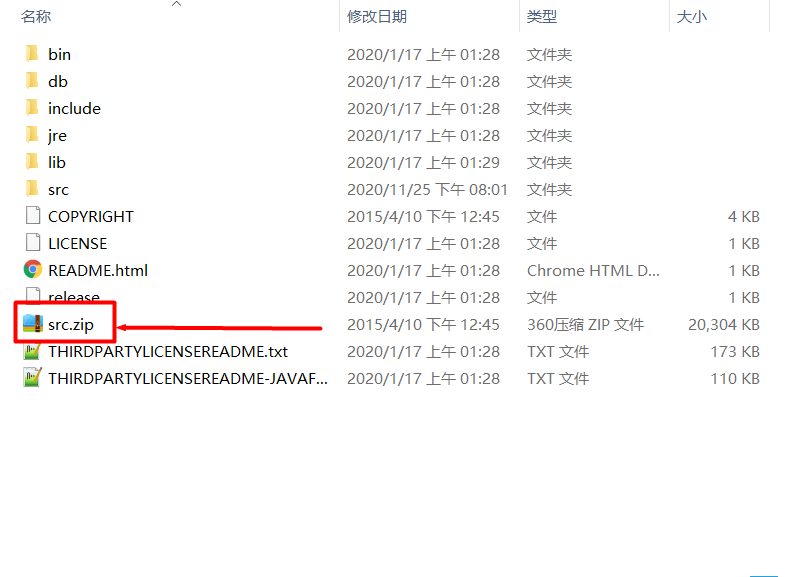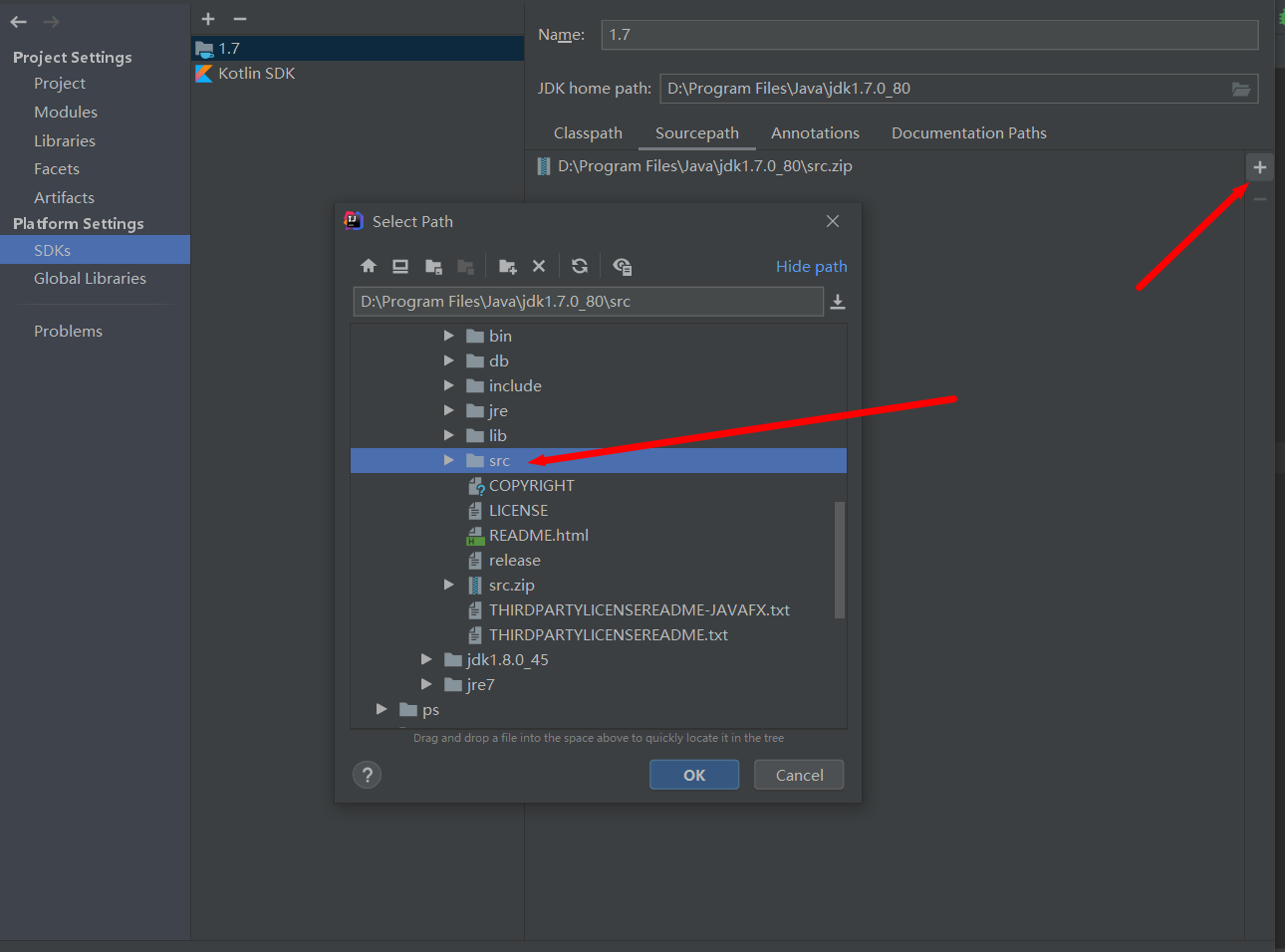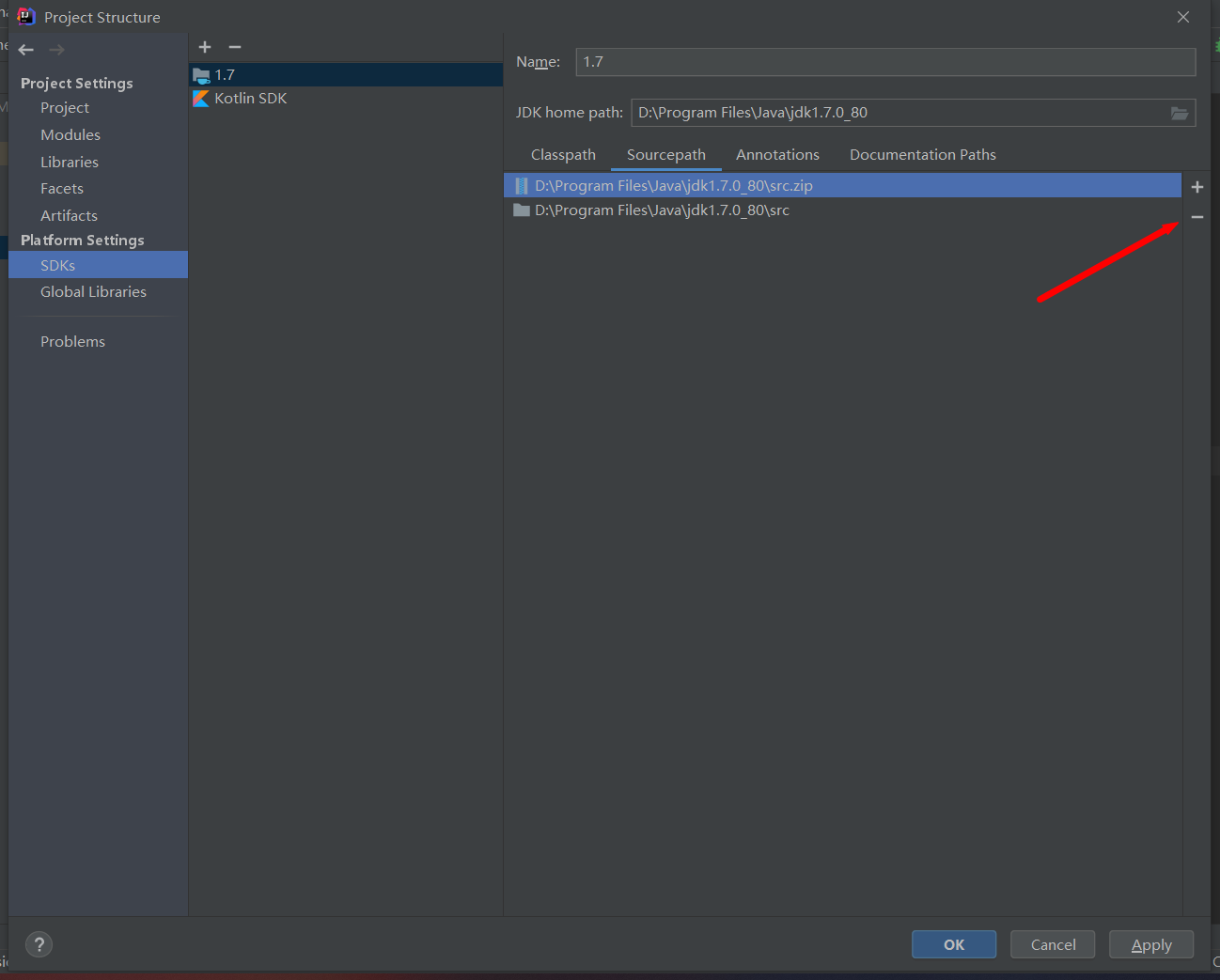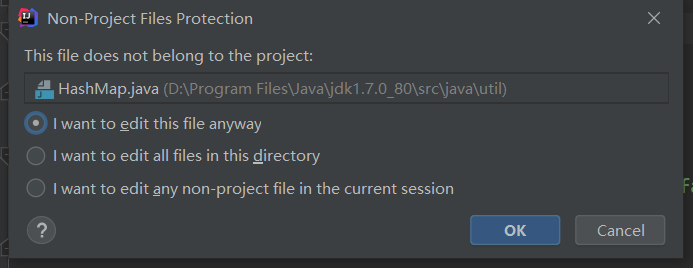public class Node{
private int number;
private Node next;
}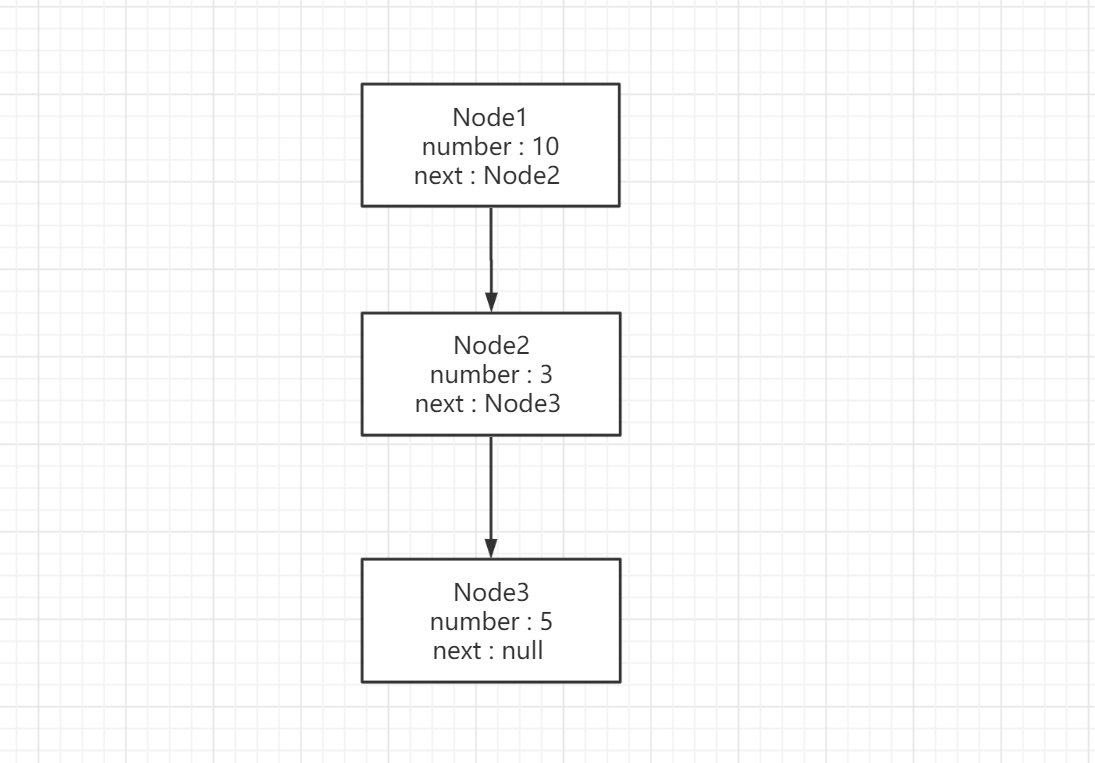JDK1.7的数据结构就是如下图所示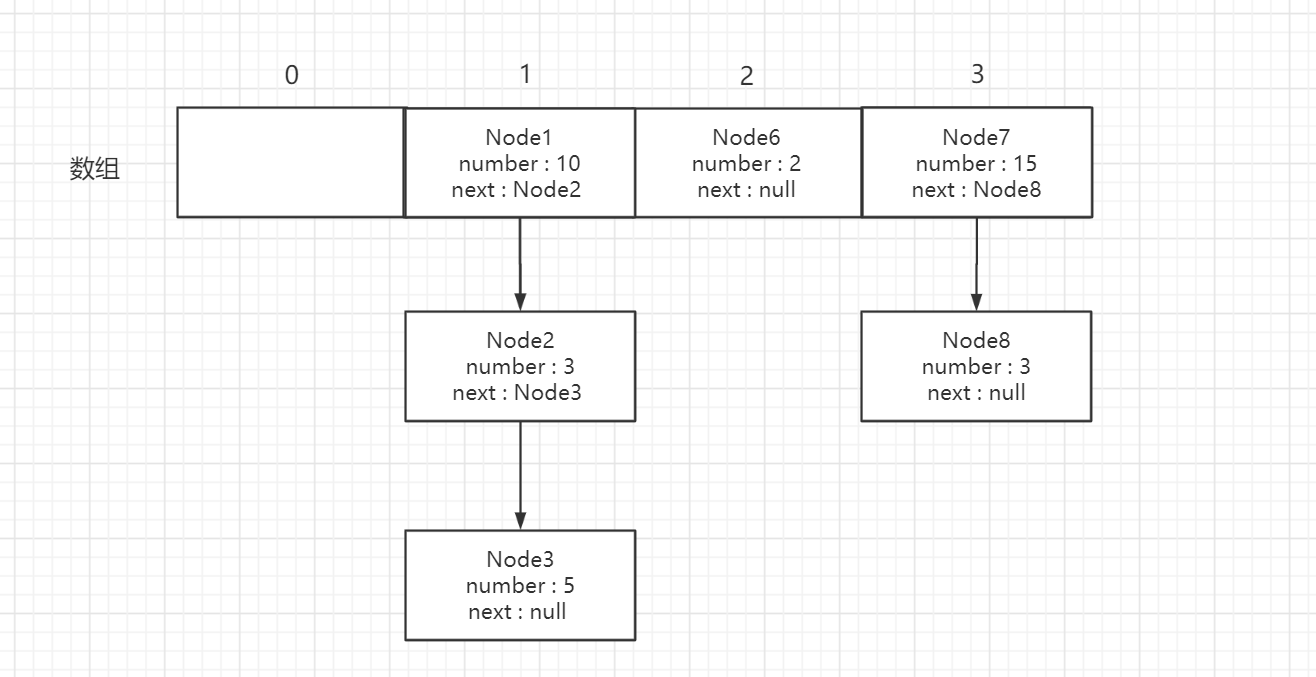## HashMap中的全局变量

static final int DEFAULT_INITIAL_CAPACITY = 1 << 4;

static final int MAXIMUM_CAPACITY = 1 << 30;

static final float DEFAULT_LOAD_FACTOR = 0.75f;

static final Entry<?, ?>[] EMPTY_TABLE = {};

transient Entry<K, V>[] table = (Entry<K, V>[]) EMPTY_TABLE;

transient int size;

int threshold;

transient int modCount;


DEFAULT_INITIAL_CAPACITY

MAXIMUM_CAPACITY

Entry[] EMPTY_TABLE

Entry<K, V>[] table = (Entry<K, V>[]) EMPTY_TABLE

size

public int size() {
return size;
}


threshold

modCount

HashMap的结构被修改的次数，用于迭代器

## 构造方法

public HashMap() {
}


public HashMap(int initialCapacity, float loadFactor) {
//初始容量不能小于0
if (initialCapacity < 0)
throw new IllegalArgumentException("Illegal initial capacity: " +
initialCapacity);
//初始容量是否大于最大容量
if (initialCapacity > MAXIMUM_CAPACITY)
//如果大于最大容量,则将容量设置为最大容量
initialCapacity = MAXIMUM_CAPACITY;
//如果负载系数小于0或者不是一个数字抛出异常
throw new IllegalArgumentException("Illegal load factor: " +
// 设置负载因子，临界值此时为容量大小，后面第一次put时由inflateTable(int toSize)方法计算设置
threshold = initialCapacity;
//空方法,由其他实现类实现
init();
}


## put方法

public V put(K key, V value) {
// 如果table引用指向成员变量EMPTY_TABLE，那么初始化HashMap（设置容量、临界值，新的Entry数组引用）
// 因为在这个对象初始化时,就是将EMPTY_TABLE这个空数组赋值给table,如果相等说明还没有进行过初始化
if (table == EMPTY_TABLE) {
inflateTable(threshold);
}
// HashMap 支持key为null
if (key == null)
//key为null单独调用存储空key的方法
return putForNullKey(value);
//计算key的hash值
int hash = hash(key);
// 根据hash值和表当前的长度，得到一个在数组中的下标,重点关注一下indexFor方法的实现。
// 该算法主要返回一个索引，0 到 table.length-1的数组下标。
int i = indexFor(hash, table.length);
//接下来，找到  table[i]处，以及该处的数据链表，看是否存在相同的key;判断key相同，
// 首先判断hash值是否相等，然后再 判断key的equals方法是否相等
for (Entry<K, V> e = table[i]; e != null; e = e.next) {
Object k;
//
//首先判断hash,并且判断如果key相等或者key的值相等那么覆盖并返回旧的value
if (e.hash == hash && ((k = e.key) == key || key.equals(k))) {
V oldValue = e.value;
e.value = value;
e.recordAccess(this);
return oldValue;
}
}

modCount++;
//进行添加操作
return null;
}


// 如果table引用指向成员变量EMPTY_TABLE，那么初始化HashMap（设置容量、临界值，新的Entry数组引用）
if (table == EMPTY_TABLE) {
inflateTable(threshold);
}


private void inflateTable(int toSize) {
// Find a power of 2 >= toSize
// 首先计算容量, toSize 容量为 threshold,在构造方法中，threshold默认等于初始容量，也就是16
int capacity = roundUpToPowerOf2(toSize);
// 然后重新计算 threshold的值，默认为 capacity * loadFactor
//Math.min 方法用于返回两个参数中的最小值
threshold = (int) Math.min(capacity * loadFactor, MAXIMUM_CAPACITY + 1);
//初始化数组 容量为 capacity
table = new Entry[capacity];
initHashSeedAsNeeded(capacity);
}


roundUpToPowerOf2方法,简单来看一下这个方法的作用

private static int roundUpToPowerOf2(int number) {
// assert number >= 0 : "number must be non-negative";
//判断参数的值是否大于最大容量
return number >= MAXIMUM_CAPACITY
//如果大于将返回最大容量
? MAXIMUM_CAPACITY
/**
* 如果小于1返回1
* highestOneBit方法可以简单理解为返回小于等于输入的数字最近的2的次方数
* 例如
* 2的1次方 2
* 2的2次方 4
* 2的3次方 8
* 2的4次方 16
* 2的5次方 32
* 小于15,并且距离15最近的2的次方数 : 8
* 小于16,并且距离16最近的2的次方数 : 16
* 小于17,并且距离17最近的2的次方数 : 16
*/
: (number > 1) ? Integer.highestOneBit((number - 1) << 1) : 1;
}


threshold = (int) Math.min(capacity * loadFactor, MAXIMUM_CAPACITY + 1);


Math.min方法是判断两个值大小,返回小的那个,如果参数具有相同的值，则结果为相同的值。如果任一值为NaN，则结果为NaN

//初始化数组 容量为 capacity
table = new Entry[capacity];


static class Entry<K, V> implements Map.Entry<K, V> {
final K key;
V value;
Entry<K, V> next;
int hash;
}


// HashMap 支持key为null
if (key == null)
//key为null单独调用存储空key的方法
return putForNullKey(value);


private V putForNullKey(V value) {
//获取下标为0的Entry节点
for (Entry<K, V> e = table; e != null; e = e.next) {
if (e.key == null) {
V oldValue = e.value;
e.value = value;
//空方法
e.recordAccess(this);
return oldValue;
}
}
modCount++;
return null;
}


void addEntry(int hash, K key, V value, int bucketIndex) {
/* JDK1.7的扩容条件；size大于等于threshold,并且新添加元素所在的索引值不等为空
也就是即使当size达到或超过threshold,新增加元素,只要不会引起hash冲突则不扩容;
JDK1.8去掉了为null的判断
*/
if ((size >= threshold) && (null != table[bucketIndex])) {
//将大小扩容到原来的两倍
resize(2 * table.length);
//如果key为null,将放到index为0的位置,否则进行取hash的操作
hash = (null != key) ? hash(key) : 0;
//根据获取的hash值进行获取下标
bucketIndex = indexFor(hash, table.length);
}
//创建entry
createEntry(hash, key, value, bucketIndex);
}


void resize(int newCapacity) {
//将旧table赋值给oldTable
Entry[] oldTable = table;
//获取旧table长度
int oldCapacity = oldTable.length;
//如果长度已经等于最大限制设置为Integer的最大值
if (oldCapacity == MAXIMUM_CAPACITY) {
threshold = Integer.MAX_VALUE;
return;
}
//创建新table,长度为参数为传入的参数newCapacity
Entry[] newTable = new Entry[newCapacity];
//该方法将oldTable的数据复制到了newTable
transfer(newTable, initHashSeedAsNeeded(newCapacity));
//将新扩容的table改为当前hashmap的存储table
table = newTable;
//重新计算阈值
threshold = (int) Math.min(newCapacity * loadFactor, MAXIMUM_CAPACITY + 1);
}


void transfer(Entry[] newTable, boolean rehash) {
//获取新创建的table长度
int newCapacity = newTable.length;
//遍历旧table
for (Entry<K, V> e : table) {
/*代码第一次判断如果当前下标entry是否为空,
如果为空则切换到下一个Entry节点
如果不为空,第二次就是判断当前下标的entry是否形成链表
如果形成链表将一直判断是否有下一个节点,当把该下标链表遍历完毕后,
然后切换到下一个entry节点进行相同的操作
* */
while (null != e) {
//获取下一个entry
Entry<K, V> next = e.next;
if (rehash) {
/**
* 判断e.key是否为null,如果为null将e.hash赋值为0
* 否则调用hash()方法进行计算hash
*/
e.hash = null == e.key ? 0 : hash(e.key);
}
//通过当前遍历旧表的entry的hash值和新table的长度来获取在新表的下标位置
int i = indexFor(e.hash, newCapacity);
/*
* jdk1.7是进行头插法,也就是不需要知道当前下标位置是否存在Entry
* 只需要将旧表中Entry节点,通过计算出下标位置
* 在新添加的Entry中直接将当前下标元素赋值给next属性,然后新添加的节点赋值给当前下标
*/
e.next = newTable[i];
newTable[i] = e;
e = next;
}
}
}


//hash()======这个方法简单理解为来通过key来计算hash,在get时通过hash可以确保是同一个entry对象
final int hash(Object k) {
int h = hashSeed;
if (0 != h && k instanceof String) {
return sun.misc.Hashing.stringHash32((String) k);
}

h ^= k.hashCode();

// This function ensures that hashCodes that differ only by
// constant multiples at each bit position have a bounded & ~
// number of collisions (approximately 8 at default load factor).
h ^= (h >>> 20) ^ (h >>> 12);
return h ^ (h >>> 7) ^ (h >>> 4);
}
//indexFor()===========
/**

0010 1101
1010 1011
结果    0010 1001
*/
static int indexFor(int h, int length) {
return h & (length - 1);
}


hash:1010 1010
15: 0000 1111

0000 1110

17通过length-1的操作为16,16的二进制为0001 0000,那么再和hash值进行&的操作
hash: 1010 1010
16: 0001 0000

JAVA开发者也想到这个问题,所以在上面的roundUpToPowerOf2()方法中进行了一些操作,防止容量不是2的次方数

void addEntry(int hash, K key, V value, int bucketIndex) {
/* JDK1.7以后的扩容条件；size大于等于threshold,并且新添加元素所在的索引值不等为空
也就是当size达到或超过threshold,新增加元素,只要不会引起hash冲突则不扩容;
JDK1.8去掉了为null的判断
*/
if ((size >= threshold) && (null != table[bucketIndex])) {
//将大小扩容到原来的两倍
resize(2 * table.length);
//如果key为null,将放到index为0的位置,否则进行取hash的操作
hash = (null != key) ? hash(key) : 0;
//根据获取的hash值进行获取下标
bucketIndex = indexFor(hash, table.length);
}
//创建entry
createEntry(hash, key, value, bucketIndex);
}


resize()方法下面取hash操作的hash()方法和获取下标的indexFor方法都已经在上面写过,这里就不再赘述

void createEntry(int hash, K key, V value, int bucketIndex) {
//先获取当前下标entry节点,也可能为null
Entry<K, V> e = table[bucketIndex];
//如果有entry节点,那么在添加新的entry时将会形成链表
table[bucketIndex] = new Entry<>(hash, key, value, e);
//将hashmap的大小加1
size++;
}


//put方法,省略一些刚刚写过的方法
int hash = hash(key);
int i = indexFor(hash, table.length);
//接下来，找到  table[i]处，以及该处的数据链表，看是否存在相同的key;判断key相同，
// 首先判断hash值是否相等，然后再 判断key的equals方法是否相等
for (Entry<K, V> e = table[i]; e != null; e = e.next) {
Object k;
//首先判断hash,如果对象的hashCode方法没有被重写,那么hash值相等两个对象一定相等
//并且判断如果key相等或者key的值相等那么覆盖并返回旧的value
if (e.hash == hash && ((k = e.key) == key || key.equals(k))) {
V oldValue = e.value;
e.value = value;
e.recordAccess(this);
return oldValue;
}
}
modCount++;
//进行添加操作
return null;


## get方法

public V get(Object key) {
//判断如果key等于null的话,直接调用得到nullkey的方法
if (key == null)
return getForNullKey();
//通过getEntry方法的到entry节点
Entry<K, V> entry = getEntry(key);
//判断如果为null返回null,否则返回entry的value
return null == entry ? null : entry.getValue();
}


private V getForNullKey() {
//如果hashmap的大小为0返回null
if (size == 0) {
return null;
}
/**
开始研究时有个问题困扰着我,写博客时突然明白了,
问题就是既然已知key为null的entry都会被放入下标0的位置,为什么还要循环,直接获取0下标的entry覆盖不行吗
然后我在写indexFor方法时想到,不仅仅为null的key下标为0,如果一个hash算法算完后通过indexFor方法
算出的下标正好是0呢,它就必须通过循环来找到那个key为null的entry
*/
for (Entry<K, V> e = table; e != null; e = e.next) {
if (e.key == null)
return e.value;
}
return null;
}


final Entry<K, V> getEntry(Object key) {
//如果hashmap的大小为0返回null
if (size == 0) {
return null;
}
//判断key如果为null则返回0,否则将key进行hash
int hash = (key == null) ? 0 : hash(key);
//indexFor方法通过hash值和table的长度获取对应的下标
//遍历该下标下的(如果有)链表
for (Entry<K, V> e = table[indexFor(hash, table.length)];
e != null;
e = e.next) {
Object k;
//判断当前entry的key的hash如果和传入的key的hash相同返回当前entry节点
if (e.hash == hash &&
((k = e.key) == key || (key != null && key.equals(k))))
return e;
}
return null;
}


## remove方法

public V remove(Object key) {
Entry<K,V> e = removeEntryForKey(key);
//如果e为null返回null,否则返回entry里面的value
return (e == null ? null : e.value);
}


final Entry<K, V> removeEntryForKey(Object key) {
if (size == 0) {
return null;
}
//取hash算index
int hash = (key == null) ? 0 : hash(key);
int i = indexFor(hash, table.length);
Entry<K, V> prev = table[i];
Entry<K, V> e = prev;
//如果当前位置不为null
while (e != null) {
Entry<K, V> next = e.next;
Object k;
//判断如果当前entry的hash相同,key相同或key的内容相同
if (e.hash == hash &&
((k = e.key) == key || (key != null && key.equals(k)))) {
modCount++;
size--;
//判断如果这个entry是链表的头结点
if (prev == e)
//将entry的下一个结点作为头结点放入数组
table[i] = next;
else
//如果当前结点不是头结点,那么就把当前entry的下一个节点替换当前entry的位置
prev.next = next;
//空方法
e.recordRemoval(this);
//返回被删除的entry节点
return e;
}
//如果没有找到对应entry节点,将继续寻找当前下标下面的entry
prev = e;
e = next;
}
//没有找到对应的entry,返回null
return e;
}


HashMap在初始化时除了一些判断数据正确性以外主要做了两件事

1. 设置负载因子大小，临界值的大小为容器容量大小

Put方法

1. 判断是否初始化过,如果没有进行过初始化,则调用inflateTable(threshold);方法进行初始化,在inflateTable方法中进行取距离参数最近的,小于参数的2的次方数,原因在上面IndexFor时也说过,然后从新计算阈值,创建新数组赋值给当前table

2. 判断key是否为null,如果为null单独调用存储key为null的方法putForNullKey在方法中进行覆盖,然后调用addEntry方法,而扩容就是在这个方法中进行的,如果不需要扩容,直接进行添加,如果需要进行扩容,则需要将这个entry的hash和所在下标位置从新计算,之后调用createEntry进行添加

3. 然后就是添加key不为null的entry,计算出hash和index,在数组中找到这个下标位置,遍历这个下标上所有的entry,如果出现重复key则覆盖,返回旧entry